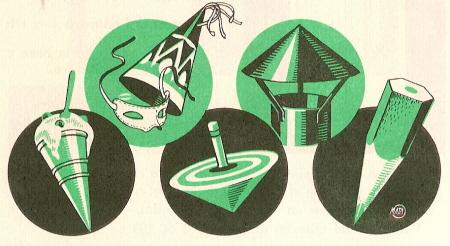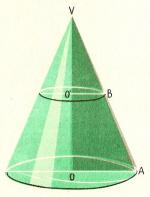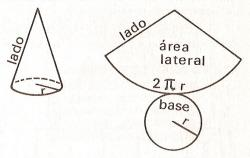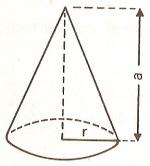Area and volume of the cone1. Cone parts

In the picture, we see several examples of cones: a clownÆs hat, the roof of a fireplace, an ice cream, a toy and a pencil point.The cone has a circular base. Its height is also the axis of symmetry. It is the perpendicular segment from the vertex to the base VO. The VA segment is the generatrix and it is called like that because when it turns, it creates the lateral surface of the cone. It is also called side.

Look at this figure and answer these questions:

 1. The segment OA is a The segment VA is a The segment VO is a2. ¢ surface and lateral area of the cone

The lateral area of a pyramid is the product of the apothem base divided by 2.
Likewise, the lateral area of the cone is the product of the length of the circumference of the base times side or generatrix divided by 2.

Lateral area of the cone = ( 2 x p x r ) x lado / 2.

The surface area is the sum of the lateral area plus the area of the circle of the base.

Surface area of the cone = lateral area + base area .

Do these problems on a paper and answer:

 1. Find the lateral area in cm2 of a cone whose generatrix or side is 4.75 cm and the radius of the base is 5 cm. 2. Find the surface area of the previous cone.. 3. A cone has as generatrix the double length than the diameter of the base, whose radius is 25 cm. What is the lateral area in cm2. ? 4. Find the surface area of the previous cone.3. Volume of the cone

Do you remember what the volume of a pyramid is? We said that the volume of the pyramid is the product of one third of the area of the base times height. In the case of the cone, its volume is equal to the product of the area of the base circle times the height divided by 3.
Volume of cone = area of base x height / 3.
Do these problems on a paper and answer:

 1. The radius of the base of a cone is 12 cm and its height is 15 cm. Find the volume in cm3. 2. The circumference of the base of a cone is 37.68 cm and 5.25 cm height. Find the volume in cm3. 3. The cone height is 14 m and the radius of the base is 7 m. Find the volume in m3. 4. The base radius of a cone is 2 m and 2.6 m height. What is its volume in m3 ?

 .adslot_S1 { display:inline-block; width: 336px; height: 280px; } @media (max-device-width:970px) { .adslot_S1 { display: none; } } If you are registered, please enter your data:   Name: Password:   | Educational applications | Mathematics | In Spanish  | To print

«Arturo Ramo GarcĒa.-Record of intellectual property of Teruel (Spain) No 141, of 29-IX-1999
Plaza Playa de Aro, 3, 1║ DO 44002-TERUEL